# Sample Question Paper (2020-21) - 2 Notes | Study Sample Papers For Class 9 - Class 9

## Class 9: Sample Question Paper (2020-21) - 2 Notes | Study Sample Papers For Class 9 - Class 9

The document Sample Question Paper (2020-21) - 2 Notes | Study Sample Papers For Class 9 - Class 9 is a part of the Class 9 Course Sample Papers For Class 9.
All you need of Class 9 at this link: Class 9

Class IX
Science
Sample Question Paper 2020-21
Max. Marks : 80
Duration : 3 hrs.

General Instructions :

1. The question paper comprises four sections A, B, C and D. There are 36 questions in the question paper. All questions are compulsory.
2. Section–A – question no. 1 to 20 - all questions and parts thereof are of one mark each. These questions contain multiple choice questions (MCQs), very short answer questions and assertion - reason type questions. Answers to these questions should be given in one word or one sentence.
3. Section–B – question no. 21 to 26 are short answer type questions, carrying 2 marks each. Answers to these questions should be in the range of 30 to 50 words.
4. Section–C – question no. 27 to 33 are short answer type questions, carrying 3 marks each. Answers to these questions should be in the range of 50 to 80 words.
5. Section–D – question no. 34 to 36 are long answer type questions carrying 5 marks each. Answer to these questions should be in the range of 80 to 120 words.
6. There is no overall choice. However, internal choices have been provided in some questions. A student has to attempt only one of the alternatives in such questions.
7. Wherever necessary, neat and properly labelled diagrams should be drawn.

Section - A

Q.1. Define a solution.  (1 Mark)
OR
What is Brownian movement?  (1 Mark)

Ans. A homogeneous mixture of two or more substances is called a solution.
OR
In a colloidal system, particles are always suspended and do not settle down. This constant colliding of the particles in continuous motion is called Brownian movement.

Q.2. Write the SI unit of weight.  (1 Mark)
Ans. SI unit of weight is Newton (N).

Q.3. A cell will swell up if   (1 Mark)
(a) the concentration of water molecules in the cell is higher than the concentration of water molecules in surrounding medium.
(b) the concentration of water molecules in surrounding medium is higher than water molecules concentration in the cell.
(c) the concentration of water molecules is same in the cell and in the surrounding medium.
(d) concentration of water molecules does not matter.
Ans. b
Solution. The medium surrounding the cell is hypotonic in nature as compare to the cell’s cytoplasm. So, in this situation water from the surrounding’s hypotonic solution (lower concentration of solute in water) is forced to enter the cell through the cell membrane due to the process of endosmosis and cause the cell to swell.

Q.4. What is the function of cutin, the waxy substance present in epidermis of desert plants?  (1 Mark)
Ans.
Cutin checks loss of water in desert plants.

Q.5. Why does the sole of the shoe wear out?  (1 Mark)
Ans. Sole of the shoe wears out due to friction between sole of the shoes and the ground.

Q.6. What is the difference between 2H and H2?  (1 Mark)
Ans. 2H means two atoms of hydrogen while, H2 means one molecule of hydrogen atom.

OR
Explain what do you understand by valence electrons.  (1 Mark)
Ans. The electrons present in the outermost shell of an atom are called as valence electrons.

Q.7. Give an example of a gas in liquid solution.  (1 Mark)
Ans.
Aerated drinks like soda water.

Q.8. Name the four elements of phloem.  (1 Mark)
Ans. Sieve tubes, companion cells, phloem parenchyma and phloem fibres.

Q.9. Atoms of most elements are not able to exist independently. Name two elements that exist as independent atoms.  (1 Mark)
Ans.
Sodium, Calcium.

OR
What are canal rays?  (1 Mark)
Ans.
The positively charged radiations produced in the discharge tube at low pressure and high voltage are called canal rays.

Q.10. Which of the following compounds have polyatomic ions; NaOH, NaCl, Na2O, NaNO3?  (1 Mark)
Ans. NaNO3.

Q.11. What are stomata?  (1 Mark)
Ans. Stomata are the small pores present in the epidermis of leaf.

OR
Name the two types of plant tissues.  (1 Mark)
Ans. Meristematic tissue and permanent tissue.

Q.12. What is uniform acceleration?  (1 Mark)
Ans. Acceleration of an object is said to be uniform if it travels in a straight line and its velocity increases or decreases (uniform retardation) by equal amounts in equal intervals of time.

OR
What indicates the motion of the earth?  (1 Mark)
Ans.
The phenomena of day and night indicate the motion of the earth.

Q.13. Given below are some measuring units of different physical quantities : kg-ms-2,  ms-2,  kg-ms-1,  ms-1,  kg-m2s-2.
Select the units which measure force and momentum respectively.   (1 Mark)
Ans. kg-ms-2, kg-m/s.

For question numbers 14, 15 and 16, two statements are given- one labelled Assertion (A) and the other labelled Reason (R). Select the correct answer to these questions from the codes (a), (b), (c) and (d) as given below:  (1 Mark)
Q.14. Assertion: Air is a mixture.
Reason: Air can be separated into its constituents like oxygen, nitrogen etc. by the physical process of fractional distillation.  (1 Mark)
(a) Both A and R are true, and R is correct explanation of the assertion.
(b) Both A and R are true, but R is not the correct explanation of the assertion.
(c) A is true, but R is false.
(d) A is false, but R is true.
Ans. a
Solution. Air is a mixture because it can be separated into its constituents like oxygen, nitrogen etc. by the physical process of fractional distillation. Also, air shows the properties of all the gases present in it.

Q.15.  For question numbers 15, two statements are given- one labelled Assertion (A) and the other labelled Reason (R). Select the correct answer to these questions from the codes (a), (b), (c) and (d) as given below:
Assertion: Husk of coconut is made of sclerenchymatous tissue.
Reason: Cells of sclerenchymatous tissue are dead with long and narrow walls thickened due to lignin.  (1 Mark)
(a) Both A and R are true, and R is correct explanation of the assertion.
(b) Both A and R are true, but R is not the correct explanation of the assertion.
(c) A is true, but R is false.
(d) A is false, but R is true.
Ans. a
Solution. Husk of coconut is made of sclerenchymatous tissue. The cells are dead with long and narrow walls thickened due to lignin.

OR
Assertion:
Muscle fibers are contractile in nature.
Reason: Cells of muscle tissue can shorten forcefully and again return to the relaxed state.  (1 Mark)
(a) Both A and R are true, and R is correct explanation of the assertion.
(b) Both A and R are true, but R is not the correct explanation of the assertion.
(c) A is true, but R is false.
(d) A is false, but R is true.
Ans. a
Solution. Muscle fibres are said to be contractile in nature because cells of muscle tissue can shorten forcefully and they can again return to the relaxed state. This specialised property is known as contractility.

Q.16. Assertion: Work done is different while climbing on stairs or using lift.
Reason: Work done depends only on the initial and final position of the object.  (1 Mark)
(a) Both A and R are true, and R is correct explanation of the assertion.
(b) Both A and R are true, but R is not the correct explanation of the assertion.
(c) A is true, but R is false.
(d) A is false, but R is true.
Ans. d
Solution. The initial and final position of the object and not on the path followed. In the above example initial and final position is same therefore work done is same in both cases.

Q. No 17 - 20 contain five sub-parts each. You are expected to answer any four sub parts in these questions.

Q.17. Read the following and answer any four questions from 17 (i) to 17 (v)
Study the given diagram and answer the following questions.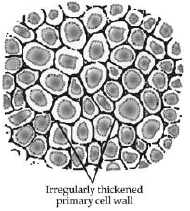(i) Identify this tissue.   (1 Mark)
(a)
Sclerenchyma.
(b) Areolar tissue.
(c) Chlorenchyma.
(d) Collenchyma.
Ans. d

(ii) Infer the characteristic features of these cells.  (1 Mark)
(a)
The cells of collenchyma are dead, circular, thickened at the corners and have large intercellular space.
(b) The cells of collenchyma are living, elongated, thickened at the corners and have very little intercellular space.
(c) The cells of collenchyma are living, elongated, very thin at the corners and have very large intercellular space.
(d) The cells of collenchyma are dead, elongated, thin at the corners and have very little intercellular space.
Ans. b

(iii) Specify the function of this tissue.  (1 Mark)
(a)
It acts as a conducting tissue.
(b) It helps mainly  in photosynthesis.
(c) It provides mechanical support to the plant.
(d) It mainly helps the plant to float the plant on water surface.
Ans. c

(iv) Name any one part of the plant where these cells are present.  (1 Mark)
(a)
It is present in roots mainly.
(b) It is present at the apex of the stem.
(c) It is present in seed coat.
(d) It is present in leaf stalk.
Ans. (d)

(v) Which of the following is not a plant tissue?  (1 Mark)
(a) Chlorenchyma
(b) Nervous tissue
(c) Xylem
(d) Phloem
Ans. (b)

Q.18. Read the following and answer any four questions from 18 (i) to 18 (v).
The following data represents the distribution of electrons, protons and neutrons in atoms of four elements A, B, C, D. Understand the data carefully and answer the following questions.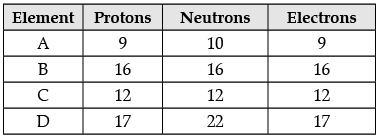(i) Give the electronic distribution of element B.  (1 Mark)
(a)
2, 8, 6
(b) 2, 8, 8
(c) 2, 6, 8
(d) 2, 8, 7
Ans. a

(ii) The valency of element A:  (1 Mark)
(a)
2
(b) 1
(c) 3
(d) 4
Ans. b

(iii) The atomic number of element B :  (1 Mark)
(a)
18
(b) 17
(c) 16
(d) 11
Ans. c

(iv) The mass number of element D :  (1 Mark)
(a)
17
(b) 22
(c) 29
(d) 39
Ans. d

(v) Number of protons in element C :  (1 Mark)
(a)
9
(b) 16
(c) 12
(d) 17
Ans. c

Q.19. Read the following and answer any four questions from 19 (i) to 19 (v)
The electronic configuration of an element ‘X’ is 2, 8, 2.

(i) The number of electrons present in the atom of element ‘X’ is  ________.  (1 Mark)
(a)
12
(b) 8
(c) 2
(d) 10
Ans. a

(ii) State True or False: The atomic number of element X is 10.  (1 Mark)
(a) True
(b) False
Ans. b

(iii) Is element ‘X’ a metal, a non-metal, metalloid, compound?  (1 Mark)

(a) ‘X’ is a compound.
(b) ‘X’ is a metalloid.
(c) ‘X’ is a metal.
(d) ‘X’ is a non-metal.
Ans. c

(iv) Find out the valency of the element ‘X’.  (1 Mark)

(a) + 4
(b) + 3
(c) + 1
(d) + 2
Ans. d

(v) Can you guess the element?  (1 Mark)
(a)
Ca
(b) Mg
(c) Fe
(d) Na
Ans. b

Q.20. Read the following and answer any 4 questions from 20 (i) to 20 (v).
(A) Seema of class IX was having common cold. She sits with Sarika who also develops the disease.
(B) Meena of class IX shifted to a new residence, with his family, where water purification system has not been installed yet. She develops cholera and dysentery.

(i) Which of these statements is correct?  (1 Mark)
(a)
Common cold is transmitted by air while cholera and dysentery by water.
(b) Common cold is transmitted by food while cholera and dysentery by air.
(c) Both of these are transmitted by air.
(d) Both of these are transmitted by water.
Ans. a

(ii) Choose the appropriate category for the above situation.  (1 Mark)
(a)
They all are non-communicable diseases.
(b) They all are communicable diseases.
(c) They all are air borne diseases.
(d) They all are water borne diseases.
Ans. b

(iii) ‘Overcrowded and poorly ventilated housing is a major factor in the spread of airborne diseases’. Choose the correct statement based on above information.   (1 Mark)
(a)
In open areas, the droplet nuclei recirculate and pose a risk to everybody majorly.
(b) When we are closer to infected person, air transmitted diseases cannot be transferred easily.
(c) When we are closer to infected person, air transmitted diseases are easily transferred
(d) All of these.
Ans. c

(iv) Why are we advised to take bland and nourishing food when we are sick?  (1 Mark)
(a)
Such food contains lot of oil or fat and spices so it is digested easily.
(b) It does not provide sufficient energy for recovery after the disease.
(c) It does not provide adequate amount of nutrients required for regeneration of cells and tissues.
(d) This food does not contain oil or fat and spices so it is digested easily and also provides sufficient energy.
Ans. d

(v)  Which of the following diseases is caused by virus?  (1 Mark)
(a) Dysentery
(b) Cholera
(c) Common cold
(d) Typhoid
Ans. c

Section - B

Q.21. Define speed and velocity. Write their SI units.  (2 Mark)
Ans. Speed is the distance travelled by an object in a given time. Its SI unit is m/s. Velocity is the speed of an object moving in a direction, or the displacement of an object in unit time. SI unit of velocity is same as speed i.e. m/s.

OR
Name the physical quantities denoted by :  (2 Mark)
(i) the slope of the distance–time graph
(ii) the area under velocity–time graph
Ans.
(i) Speed
(ii) Displacement

Q.22. The kinetic energy of an object moving with a velocity of 5 m/s is 25 J. Find the mass of the object. What will be its kinetic energy when its velocity is made two times?  (2 Mark)
Ans. Mass = m, v = 5 m/s, K.E. = 25 J
K.E. = 1/2 mv2
m = 25 x 2/25 = 2 kg
K.E. = 1/2 × 2 × (10)2 = 100 J.

Q.23. A solution contains 60 g of common salt in 240 g of water. Calculate the concentration in terms of mass by mass percentage of solution.  (2 Mark)
Ans.
Concentration of solution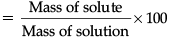Mass of common salt is 60 g.

Mass of water is 240 g.

Mass of solution = (60 + 240)g = 300 g.

Concentration of solution =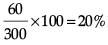OR
Give the principle of the following technique used in separation of mixture : (i) Centrifugation (ii) Chromatography  (2 Mark)
Ans. (i) Centrifugation : Two components having difference in densities can be separated by centrifugation. When the mixture is rotated fast, the lighter particles come to the top and the heavier remain at the bottom.
(ii) Chromatography : This method is used to separate a mixture of different dyes. It is based on the difference in solubilities of different solutes in the same solvent.

Q.24. What are isotopes? Write three isotopes of hydrogen.  (2 Mark)
Ans.
Isotopes : Isotopes are the atoms of the same element having same atomic number, but different mass numbers.
Three isotopes of hydrogen 1H1, 1H2, 1H3

Q.25. State two ways by which you can change a saturated solution to unsaturated solution.  (2 Mark)
Ans. (i) By increasing the temperature by heating the solution.
(ii) By increasing the amount of solvent.

Q.26. Two elements 1H1 and 16O8 form a compound ‘Z’, which is required during the photosynthesis by plants and is necessary for life.
(i) Identify ‘Z’.
(ii) Write its molar mass.   (2 Mark)

Ans. (i) Water
(ii) Molar mass of H2O = 2 × 1 + 16 = 18 g mol–1

Section - C

Q.27. (i) What is the relation between the mass m and the weight w of a body?
(ii) What are the differences between mass and weight?   (3 Mark)
Ans.
(i) Weight of a body is the force of attraction of the earth on that body. This force depends on the mass (m) of the body and the acceleration due to gravity (g).
F = m × a
F = m × g
W = m × g
The weight (W) of the body is directly proportional to the mass of the body.
(ii)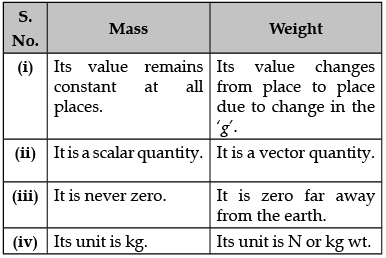OR
Find the weight of an object at a height 6400 km above the earth’s surface. The weight of the object at the surface of the earth is 20 N and the radius of the earth is 6,400 km.   (3 Mark)

Ans. W = mg = 20 N
Mass = 20/9.8 = 2.04 kg, g = GMe / Re2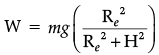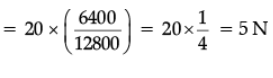Q.28. A person was bitten by a stray dog. After some days his nature gets irritated, he started fearing water :
(i) Name the disease.
(ii) Is there any vaccine available?
(iii) Is there any plan of your local authority for the control of this disease?  (3 Mark)
Ans.
(i) Rabies.
(ii) Anti-rabies vaccine is available.
(iii) Compulsory immunization of pet / dogs with anti-rabies vaccine is available with local authority.

Q.29. Identify the type of tissues in the following :  (3 Mark)
(i) Vascular bundle
(ii) Inner lining of the intestine
(iii) Lining of kidney tubule
(iv) Iris of the eye
(v) Muscles of the heart
(vi) Bronchi of lungs.

Ans. (i) Complex tissues
(ii) Columnar epithelium
(iii) Cuboidal epithelium
(iv) Involuntary muscular tissues
(v) Cardiac muscles
(vi) Ciliated cuboidal epithelium.

Q.30. (a) What is the relationship between two elements X and Y whose atomic numbers are 18 and 20 respectively, but their mass numbers remain same as 40 ?
(b) Which has more number of electrons : Na or Na+ ? Why ?
(c) Name the isotope used to treat  : (i) Goitre (ii) Cancer   (3 Mark)
Ans.
(a) Isobars. (X-Argon and Y-Calcium)
(b) Na contains one electron more than Na+. The (+) ve charge indicates that it lost one electron.
(c) (i) Isotope of iodine is used in the treatment of goitre.
(ii) Isotope of cobalt is used in the treatment of cancer.

Q.31. A ball is allowed to roll down from an inclined plane. It reaches the foot of the plane and continues to roll on the ground. It stops after travelling some distance. Is this the violation of law of inertia? Give reasons for your answer.  (3 Mark)
Ans.
(i) No, It is not the violation of law of inertia. Law of inertia is obeyed only when no external force acts on a body. But in this case the friction due to the ground acts on the ball, so it comes to rest.
(ii) Player lowers his hand because by doing so he increases the time in which velocity of ball comes to zero. This decreases the rate of change of momentum and so the impact of force is reduced.

Q.32. (i) Write one point of difference between concentration and solubility.
(ii) What is the effect of temperature on the rate of solubility? (3 Mark)
Ans.
(i) Concentration of solution is the amount of solute present in a given amount of solution or the amount of solute dissolved in a given mass or volume of the solvent. Solubility is the maximum amount of solute that can be dissolved in a given solution at a given temperature.
(ii) The rate of solubility increases with increase in temperature.

Q.33. Give the names of the elements present in the following compounds.
(a) Quick lime
(b) Hydrogen bromide
(c) Baking powder  (3 Mark)
Ans. (a) Calcium and oxygen
(b) Hydrogen and bromine
(c) Sodium, hydrogen, carbon and oxygen

Section - D

Q.34. (a) What is the name given to the thread shaped structures in the nucleus? Why is it important ?
(b) Draw a diagram of the nucleus to show the given parts : (i) nucleolus (ii) nuclear pore
(iii) nuclear envelope  (5 Mark)

Ans. (a) The thread shaped structures in the nucleus are known as chromosomes. These are important because they contain information for inheritance of features from parents to the next generation.
(b)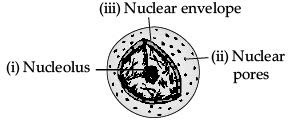OR
(a) What kind of food is advised when we fall sick and why?
(b) Mention any three basic conditions required for good health.  (5 Mark)
Ans. (a) (i) We should take bland and nourishing food. Such food does not contain fat, oil or spices, so digested easily.
(ii) It provides sufficient energy and nutrients which are required for recovery and regeneration.
(b) (i) Balanced diet
(ii) Personal hygiene
(iii) Clean surrounding
(iv) Clean food and water
(v) Clean air
(vi) Exercises and relaxation
(viii) Good economic condition

Q.35. Explain Rutherford’s a-particles scattering experiment with diagram and observations.   (5 Mark)
Ans. Rutherford bombarded a stream of α-particles on a thin gold foil.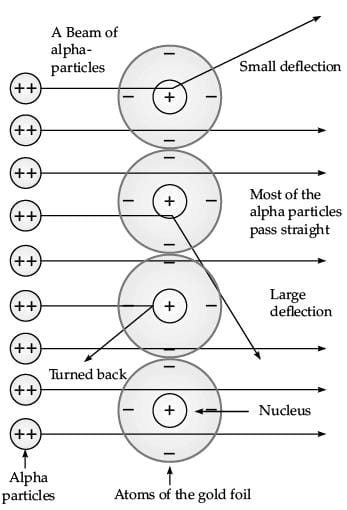(i) Most of the α-particles passed through the foil without any deflection.
(ii) A few α-particles were deflected through small angles and few through larger angles.
(iii) The number of α-particles that bounced back was very small.
The important conclusions drawn from the experiment are :
(i) An atom consists of positively charged centre called nucleus.
(ii) The electrons revolve around the nucleus in well defined orbits.
(iii) The size of the nucleus is very small as compared to the size of the atom.

Q.36.  (i) A sample of vitamin C is known to contain 2.58 × 1024 oxygen atoms. How many moles of oxygen atoms are present in the sample?
(ii) Write one word for the following :
(a) In a balanced chemical equation, the sum of the masses of reactants and products remains unchanged.
(b) A group of atoms carrying a fixed charge on them.
(iii) Write chemical formulae of the following compounds :
(a) Sodium phosphate
(b) Ammonium carbonate   (5 Mark)

Ans. (i) Number of moles = Given no. of particles / Avogadro number

= 2.58 x 1024/6.022 x 1023

= 0.4284 × 10

= 4.284 moles.

(ii) (a) Law of conservation of mass.

(b) Ion

(iii) (a) Na3PO4

(b) (NH4)2CO3

OR
(i) State the law of conservation of mass. In a chemical reaction 4.2 g of sodium hydrogen carbonate reacted with 3.0 g of ethanoic acid. The products obtained were 4.1 g of sodium ethanoate, 0.9 g of water and 2.2 g of carbon dioxide. Show that these observations are in agreement with the law of conservation of mass.
(ii) Define molecular mass. Find the relative molecular mass of calcium chloride. (Given Ca = 40 u ; Cl = 35.5 u)   (5 Mark)

Ans. (i) Mass can neither be created nor destroyed in a chemical reaction.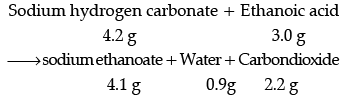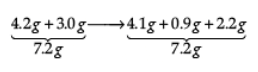These observations are in agreement with the law of conservation of mass because in a chemical reaction total mass of reactants is equal to total mass of the products.
(ii) The molecular mass of a substance is the sum of the atomic masses of all the atoms in a molecule of the substance.
Molecular mass of calcium chloride
CaCl2 = 40 + 35.5 × 2 = 111.0 u

The document Sample Question Paper (2020-21) - 2 Notes | Study Sample Papers For Class 9 - Class 9 is a part of the Class 9 Course Sample Papers For Class 9.
All you need of Class 9 at this link: Class 9Use Code STAYHOME200 and get INR 200 additional OFF

## Sample Papers For Class 9

191 docs|12 tests

Track your progress, build streaks, highlight & save important lessons and more!

,

,

,

,

,

,

,

,

,

,

,

,

,

,

,

,

,

,

,

,

,

;